61. Suppose a processor does not have any stack pointer register. Which of the following statements is true? a. It cannot have subroutine call instruction b. It can have subroutine call instruction, but no nested subroutine calls c. Nested subroutine calls are possible, but interrupts are not d. All sequences of subroutine calls and also interrupts are possible
 Answer: (a).It cannot have subroutine call instruction

 62. Given the following Karnaugh map, which one of the following represents the minimal Sum-Of-Products of the map?a. xy + y'z b. wx'y' + xy + xz c. w'x + y'z + xy d. xz + y

 63. A processor needs software interrupt to a. test the interrupt system of the processor b. implement co-routines c. obtain system services which need execution of privileged instructions d. return from subroutine
 Answer: (c).obtain system services which need execution of privileged instructions

 64. A CPU has two modes-privileged and non-privileged. In order to change the mode from privileged to non-privileged a. a hardware interrupt is needed b. a software interrupt is needed c. a privileged instruction (which does not generate an interrupt) is needed d. a non-privileged instruction (which does not generate an interrupt is needed
 Answer: (b).a software interrupt is needed

 65. Consider the following circuit with initial state Q0 = Q1 = 0. The D Flip-flops are positive edged triggered and have set up times 20 nanosecond and hold times 0. Also, consider the following timing diagrams of X and C; the clock period of C <= 40 nanosecond. Which one is the correct plot of Y?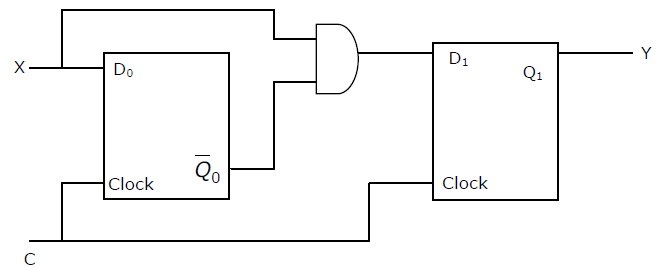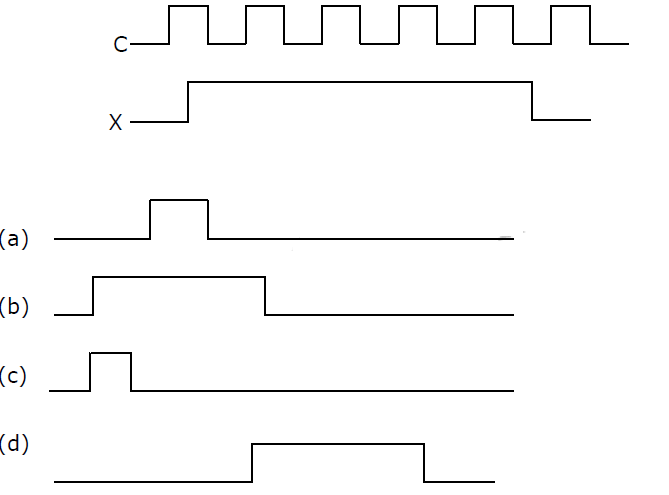a. a b. b c. c d. d

 66. Which is the most appropriate match for the items in the first column with the items in the second column:X. Indirect Addressing I. Array implementationY. Indexed Addressing II. Writing re-locatable codeZ. Base Register Addressing III. Passing array as parameter a. (X, III) (Y, I) (Z, II) b. (X, II) (Y, III) (Z, I) c. (X, III) (Y, II) (Z, I) d. (X, I) (Y, III) (Z, II)
 Answer: (a).(X, III) (Y, I) (Z, II)

 67. The 2’s complement representation of (−539)10 in hexadecimal is a. ABE b. DBC c. DE5 d. 9E7
 68. Consider the circuit shown below. The output of a 2:1 Mux is given by the function (ac' + bc). Which of the following is true?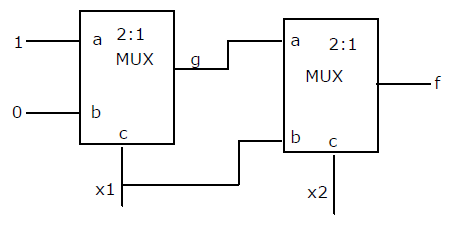a. f = x1'+ x1x b. f = x1'x2 + x1x2' c. f = x1x2 + x1'x2' d. f = x1 + x2'
 69. Consider the circuit given below with initial state Q0 =1, Q1 = Q2 = 0. The state of the circuit is given by the value 4Q2 + 2Q1 + Q0.Which one of the following is the correct state sequence of the circuit?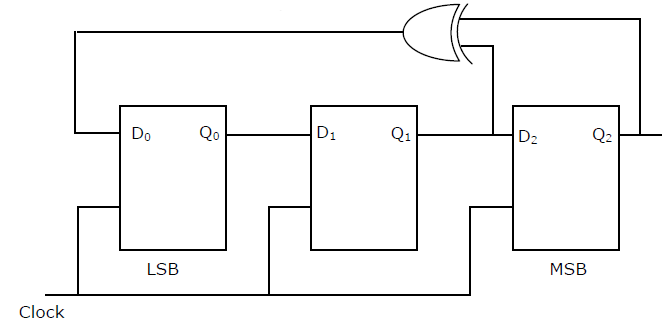a. 1,3,4,6,7,5,2 b. 1,2,5,3,7,6,4 c. 1,2,7,3,5,6,4 d. 1,6,5,7,2,3,4
 70. Consider the following data path of a simple non-pilelined CPU. The registers A, B, A1, A2, MDR, the bus and the ALU are 8-bit wide. SP and MAR are 16-bit registers. The MUX is of size 8 × (2:1) and the DEMUX is of size 8 × (1:2). Each memory operation takes 2 CPU clock cycles and uses MAR (Memory Address Register) and MDR (Memory Date Register). SP can be decremented locally.The CPU instruction “push r”, where = A or B, has the specificationM [SP]How many CPU clock cycles are needed to execute the “push r” instruction?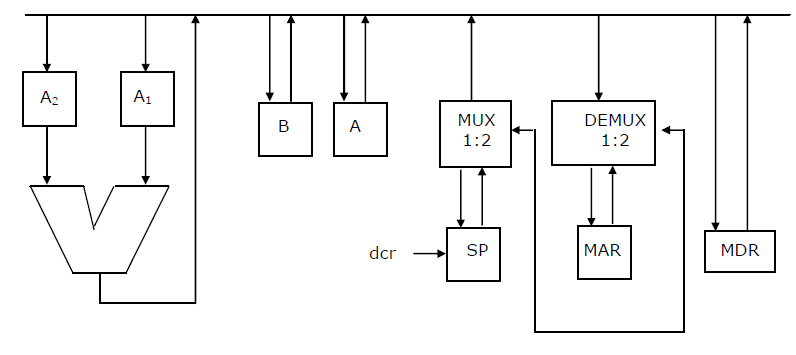a. 1 b. 3 c. 4 d. 5